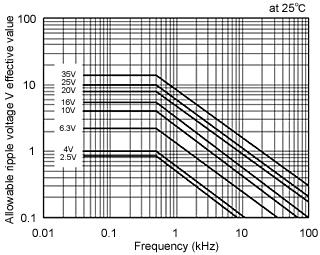FAQ's
 What are the allowable levels for ripple voltage and current? The allowable ripple voltage must take a sine wave shape (see below). For other waveform types, please contact a ROHM representative.The permissible temperature increase due to ripple voltage/current is 5ºC max. At temperatures above this level the dielectric will begin to deteriorate, resulting in possible short-circuits.In order to determine the limits for the allowable ripple voltage at high temperatures, please refer to the following formulas: E=allowable ripple voltageE Max.(at50ºC)=0.7×E Max.(at 25ºC)E Max.(at85ºC)=0.5×E Max.(at 25ºC)E Max.(at125ºC)=0.3×E Max.(at 25ºC)